# Constructions Class 9 Notes: Chapter 11

According to the CBSE Syllabus 2023-24, this chapter has been removed from NCERT Class 9 Maths textbook.

## Introduction to Constructions

### Linear Pair axiom

• If a ray stands on a line then the adjacent angles form a linear pair of angles.
• If two angles form a linear pair, then uncommon arms of both angles form a straight line.

## Angle Bisector### Construction of an Angle bisector

Suppose we want to draw the angle bisector of ∠ABC we will do it as follows:

• Taking B as the centre and any radius, draw an arc to intersect AB and BC to intersect at D and E, respectively.
• Taking D and E as centres and with a radius of more than DE/2, draw arcs to intersect each other at a point F.
• Draw the ray BF. This ray BF is the required bisector of the ∠ABC.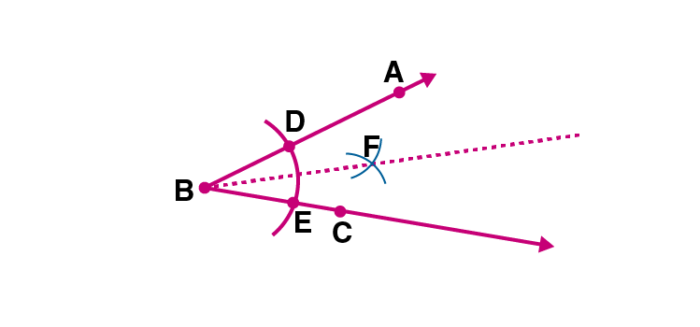To know more about Angle Bisector, visit here.

## Perpendicular Bisector

### Construction of a perpendicular bisector

Steps of construction of a perpendicular bisector on the line segment AB:

• Take A and B as centres and radii more than AB/2 draw arcs on both sides of the line.
• Arcs intersect at points C and D. Join CD.
• CD intersects AB at M. CMD is the required perpendicular bisector of the line segment AB.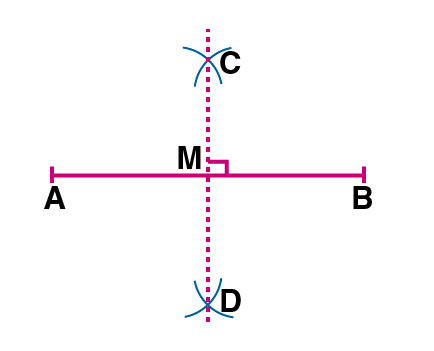### Proof of the validity of construction of a perpendicular bisector

Proof of the validity of the construction of the perpendicular bisector:
ΔDAC and ΔDBC are congruent by SSS congruency. (∵ AC = BC, AD = BD and CD = CD)
∠ACM and ∠BCMare equal (cpct)
ΔAMC and ΔBMC are congruent by SAS congruency. (∵ AC = BC, ∠ACM = ∠BCM and CM = CM)
AM = BM and ∠AMC = ∠BMC (CPCT)
∠AMC + ∠BMC = 1800 (Linear Pair Axiom)
∴ ∠AMC = ∠BMC = 90
Therefore, CMD is the perpendicular bisector.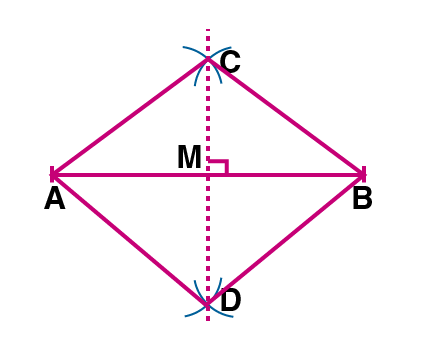To know more about Perpendicular Bisector, visit here.

## Constructing Angles

### Construction of an Angle of 60 Degrees

Steps of construction of an angle of 60 degrees:

• Draw a ray QR.
• Take Q as the centre and some radius draw an arc of a circle, which intersects QR at a point Y.
• Take Y as the centre with the same radius; draw an arc intersecting the previously drawn arc at point X.
• Draw a ray QP passing through X
• ∠PQR=60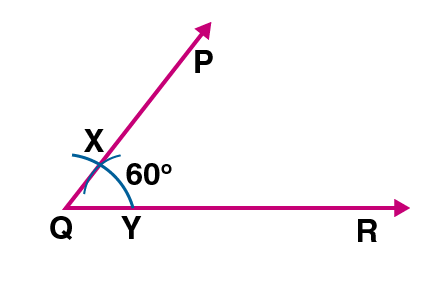### Proof for the validity of construction of an angle of 60 degrees

Proof for the validity of construction of the 600 angle:
Join XY
XY = XQ = YQ (By construction)
∴△XQY is an equilateral triangle.
Therefore, ∠XQY = ∠PQR = 600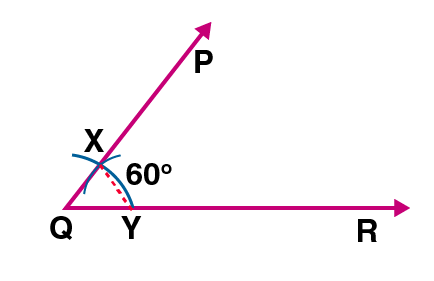To know more about Constructing Angles, visit here.

## Triangle Constructions

### Construction of Triangles

At least three parts of a triangle have to be given for constructing it, but not all combinations of three parts are sufficient for the purpose.
Therefore a unique triangle can be constructed if the  following parts of a triangle are given:

• Two sides and the included angle is given.
• Three sides are given.
• Two angles and the included side is given.
• In a right triangle, a hypotenuse and one side are given.
• If two sides and an angle (not the included angle) are given, it is not always possible to construct such a triangle uniquely.

### Given base, base angle and the sum of other two sides

Steps for construction of a triangle given base, base angle, and the sum of the other two sides:

• Draw the base BC and at point B make an angle say XBC, equal to the given angle.
• Cut the line segment BD equal to AB + AC from ray BX.
• Join DC and make an angle DCY equal to ∠BDC.
• Let CY intersect BX at A.
• ABC is the required triangle.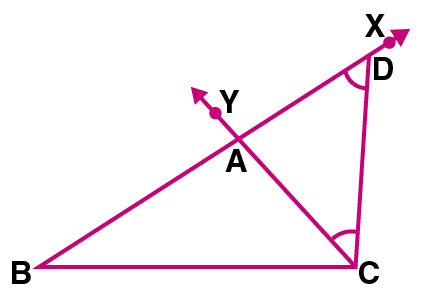### Given base(BC), base angle(ABC) and AB-AC

Steps of construction of a triangle given base(BC), base angle(∠ABC) and difference of the other two sides (AB-AC):

• Draw base BC and with point B as the vertex make an angle XBC equal to the given angle.
• Cut the line segment BD equal to AB – AC(AB > AC) on the ray BX.
• Join DC and draw the perpendicular bisector PQ of DC.
• Let it intersect BX at a point A. Join AC.
• Then △ABC is the required triangle.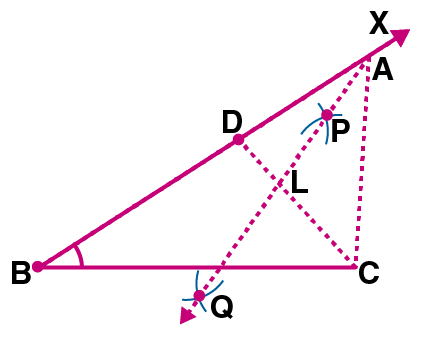### Proof for validation for construction of a triangle with given base, base angle and difference between two sides

Validation of the steps of construction of a triangle with given base, base angle and difference between two sides

• Base BC and ∠B are drawn as given.
• Point A lies on the perpendicular bisector of DC. So, AD = AC.
• BD = AB – AD = AB – AC (∵ AD = AC).
• Therefore ABC is the required triangle.### Given base (BC), base angle (ABC) and AC-AB

Steps of construction of a triangle given base (BC), base angle (∠ABC) and difference of the other two sides (AC-AB):

• Draw the base BC and at point B make an angle XBC equal to the given angle.
• Cut the line segment BD equal to AC – AB from the line BX extended on the opposite side of line segment BC.
• Join DC and draw the perpendicular bisector, say PQ of DC.
• Let PQ intersect BX at A. Join AC.
• △ABC is the required triangle.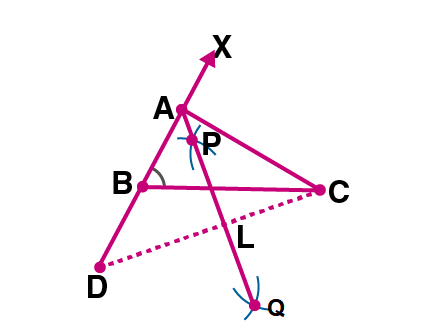### Given perimeter and two base angles

Steps of construction of a triangle with a given perimeter and two base angles.

• Draw a line segment, say GH equal to BC + CA + AB.
• Make angles XGH equal to ∠B and YHG equal to ∠C, where angle B and C are the given base angles.
• Draw the angle bisector of∠XGH and ∠YHG. Let these bisectors intersect at a point A.
• Draw perpendicular bisectors PQ of AG and RS of AH.
• Let PQ intersect GH at B and RS intersect GH at C. Join AB and AC
• △ABC is the required triangle.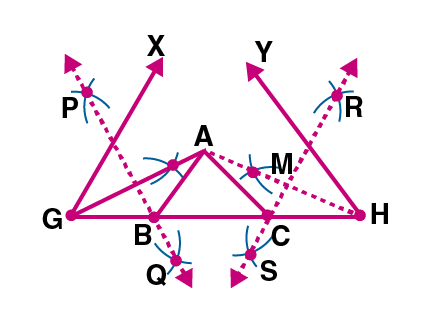To know more about Triangle Constructions, visit here.

### Proof for validation for the construction of a triangle with a given perimeter and two base angles

Validating the steps of construction of a triangle with a given perimeter and two base angles:

• B lies on the perpendicular bisector PQ of AG and C lies on the perpendicular bisector RS of AH. So, GB = AB and CH = AC.
• BC + CA + AB = BC + GB + CH = GH (∵ GB = AB and CH = AC)
• ∠BAG = ∠AGB (∵ΔAGB, AB = GB)
• ∠ABC = ∠BAG + ∠AGB = 2∠AGB = ∠XGH
• Similarly, ∠ACB =∠YHG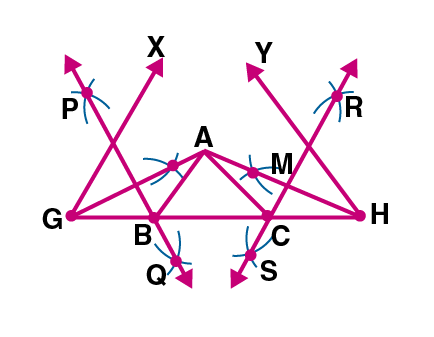## Frequently Asked Questions on CBSE Class 9 Maths Notes Chapter 11: Construction

Q1

### What is an angle bisector?

Angle bisector is the line or line segment that divides the angle into two equal parts.

Q2

### What is the Base angle theorem?

The base angles theorem states that if the sides of a triangle are congruent (Isosceles triangle), then the angles opposite these sides are congruent.

Q3

### What is a Perpendicular theorem?

A perpendicular bisector is a line that bisects another line segment at a right angle, through the intersection point.